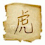## Friday, December 12, 2014

### 52 Things: Number 10: What is the difference between the RSA and strong-RSA problem?

This is the latest in a series of blog posts to address the list of '52 Things Every PhD Student Should Know To Do Cryptography': a set of questions compiled to give PhD candidates a sense of what they should know by the end of their first year. This blog post introduces the RSA and Strong-RSA problems and highlights the differences between the two.

Cryptography relies heavily on the assumption that certain mathematical problems are hard to solve in a realistic amount of time. When looking at Public-Key (Asymmetric) Cryptography, which is what we'll be focusing on in this blog post we use the assumed existence of One-Way functions, i.e. functions that are easy to compute one way but are difficult to invert. We use problems from algorithmic number theory to produce these functions.

Factoring

The first difficult problem from number theory to talk about is factoring. Given a composite integer $N$ the factoring problem is to find positive integers $p,q$ such that $N = pq$. Although on the face of it this seems like a very simple problem, this is in fact a very tough, well studied problem. This can be solved in exponential time by checking all the numbers $p = 2, \ldots, \sqrt{N}$. However, solving a problem in exponential time is not fast enough. No polynomial time algorithm has been developed to solve the factoring problem, despite many years of research. Clearly there are examples of $N$ for which this is very easy to solve, for example whenever $N$ is even. Therefore, when starting to think about using this in a Cryptographic construction we consider $N$ as very large and being constructed by 2 large primes $p,q$.

The RSA Problem

In RSA public-key encryption  Alice encrypts a plaintext $M$ using Bob's public key $(n,e)$ to ciphertext $C$ by $C = M^e (\textrm{mod } n)$ where $n$ is the product of two large primes and $e \geq 3$ is an odd integer that is coprime to the order of $\mathbb{Z}_n^{*}$, the group of invertible elements of $\mathbb{Z}_n$. Bob knows the private key $(n,d)$ where $de = 1 (\textrm{ mod } (p-1)(q-1))$ meaning he can compute $M = C^d (\textrm{mod } n)$. An adversary can eavesdrop $C$ and can know the public key $(n, e)$ however to calculate $M$ the adversary must find the factors of $n$. Therefore, this means the RSA problem is no harder than integer factorisation but is still a very hard problem to solve provided a suitable $n$ is chosen.

The Strong RSA Assumption

The strong RSA assumption differs from the RSA assumption in that the adversary can choose the (odd) public exponent $e \geq 3$. The adversary's task is to compute the plaintext $M$ from the ciphertext given that $C = M^e (\textrm{mod } n)$. This is at least as easy as the RSA problem meaning that the strong RSA assumption is, unsurprisingly, a stronger assumption. The RSA problem is now over a quarter of a century old. Public key encryption schemes have been developed that derive their strength fully from the RSA problem.

1.1.